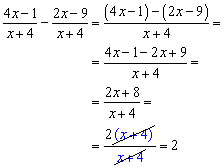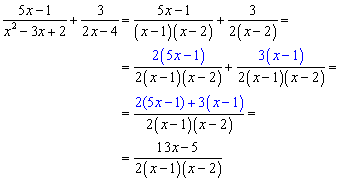# Week 15- Adding and Subtracting of Rational Expression

This week, I have learned how to add and subtract rational expression.First, as it is similar to fractions, you must make the denominator the same

Second, we multiply the top number with the opposite denominator. In this picture, since it has the same denominator, you can add or subtract right away.

Finally, you simplify. If you see something that are the same, you can cross them out to get the answer.

Let’s try a harder question.First we factor so that we can see what are the possibility of the same denominator.

Second, you take the number which can have the same denominator.